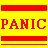#MegaEpix Enormouspresents (with help from our friends @) MEMBRANE.COM

## Vortices Formulae

velocity = distance/time

acceleration = velocity/time

forced vortex:
circulation = tau = 2wA
where w = rotation = average angular velocity (a vector quantity)
A = area enclosed by the contour

free vortex:
circulation = 2(pi)C

## Vortex Prose

Vortices
Vortex flow

Vortices II
Vortices and singularites

Vortices III
A historical note

Cartesian Vortices
Musings by Rene Descartes

Cartesian Vortices: Yabuttal
a Yabuttal for Rene

The Decline of Determinism
The first blow: The Uncertainty Principle

## Example Problem Where Earth is in a Vortex

if acceleration = velocity/time
and v = speed of light = 2.9979*10^8 m/s
and for the sake of argument we are accelerating at pi (rounded to 15 decimal places) m/s^2

then time = 95426120.77903860 seconds (rounded to 16 significant digits)
= 1104.468990498130 days
= 3.025942439720910 years = roughly 3 years + 9.5 days as relative to the rotation and revolution of the planet earth.

a couple other fundamental physical constants to contemplate:
permitivity of free space = 8.854*10^(-12) C^2/(N*m)
permeability of free space = 4(pi)*10^(-7) Wb/(A*m)

SIDD:

the tricky part is .. u cannot maintain a constant acceleration like pi m/s^2 on a massive body

Does anyone want details ?